Diffuse scattering: Stacking faultsDiffuse scattering
Thermal I
Thermal II
Occupational I
Occupational II
Longitudinal waves
Transversal waves
Short range order
Stacking faults

Interactive examples
Displacement waves
Short range order
Stacking faults

Goto
Contents

This example shows the effect of stacking faults. They are illustrated by use of a 2-D example. The crystal is build up of rows corresponding to a primitive unit structure. Three different layers are stacked: a at x=0.0, b at x=1/3 and c at x=2/3. The stacking sequence is controlled by two probabilities ALPHA and BETA. The meaning of these probabilities is defined as:

 ``` ALPHA probability of "ab" followed by "a" 1 - ALPHA probability of "ab" followed by "c" BETA probability of "ba" followed by "b" 1 - BETA probability of "ba" followed by "c"``` ``` ALPHA BETA resulting structure 0.0 0.0 pure cubic sequence 0.05 0.05 Sequence of cubic twins 0.5 0.5 random stacking 1.0 1.0 pure hexagonal sequence```

The layers "a","b" and "c" can be rotated cylindrically. The following images show a sequence going from ALPHA=BETA=0, i.e. pure cubic sequence to ALPHA=BETA=1, a pure hexagonal sequence. The left image shows part of the crystal, the right image the corresponding Fourier transform. To get a larger image just click on the corresponding picture.

A note on the indices: The crystal was created on a square coordinate system with the x=1 being the distance between atoms in each row and y=1 being the separation of rows. Accordingly the reciprocal space direction labeled corresponds to the  direction of the three dimensional hexagonal or cubic crystal. The reciprocal k direction corresponds to the hexagonal  and the cubic  direction. The spacing is doubled for a hexagonal crystal and tripled for a cubic crystal.

ALPHA=BETA=0.00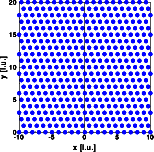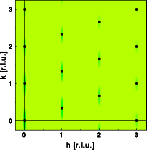The first structure is a perfect "abc" sequence. Only sharp Bragg reflections of the cubic structure are observed.

ALPHA=BETA=0.05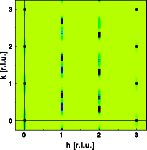The probability of a hexagonal sequence is very low. Accordingly if such a sequence occurs, the crystal is continued immediately as the cubic structure in its twined orientation. The Bragg reflections at h=0 and h=3 remain invariant, in addition to the Bragg reflections of the first image, those of the twined orientation appear. Weak diffuse streaks connect the Bragg reflections at h=1 and h=2.

ALPHA=BETA=0.20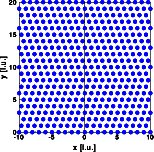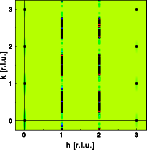The probability for hexagonal stacking increases. The Bragg reflections at h=1 and h=2 are replaced by diffuse streaks that are absent at integer k.

ALPHA=BETA=0.50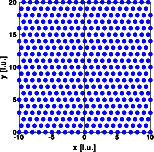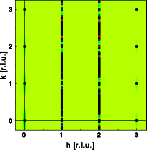The random stacking of cubic and hexagonal sequences causes continuous diffuse streaks at h=1 and h=3. As for all the other situations, the Bragg reflections at h=3n remain invariant.

ALPHA=BETA=0.80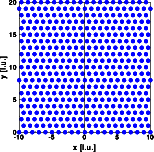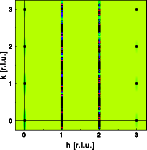The crystal becomes predominantly hexagonal. The diffuse streaks start to develop sharp maxima at k=n and k=n+0.5.

ALPHA=BETA=1.00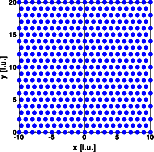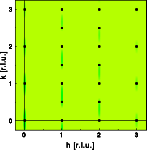A perfect hexagonal crystal has been created. The diffuse streaks have disappeared, the Bragg reflections of the hexagonal structure result.
© Th. Proffen and R.B. Neder, 2003